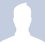# Just how many coin tosses would it take?I recently came in pocession of a fereign currency coin which seemed to be biased(to heads say). On noticing that the coin seemed asymmetric, I set up the experiment and started tossing the coin. Here is how things went :

after 2 coin tosses: 2H, 0T : Prob(H) =1

after 30 coin tosses: 15H, 15T : Prob(H) = .5

after 3000 coin tosses : 1492H, 1508T : Prob(H) = .497333333333333333333333333333

Now to state the obvious, the first result was a joke. In the third case, notice how I've conveniently written the number to more than 10 places of decimal. At some point, I had to stop and think, does it make any sense to claim the number to 10 places of decimal with just 3000 observations?

One thing I could agree upon was that, higher the number of observations, higher is the precision, but how much?

I guess I want to quantify precision as a function of number of observations.

Please share your thoughts on the problem, any suggestion would be appreciated.Note by Rahul Dewani
5 years, 3 months ago

This discussion board is a place to discuss our Daily Challenges and the math and science related to those challenges. Explanations are more than just a solution — they should explain the steps and thinking strategies that you used to obtain the solution. Comments should further the discussion of math and science.

When posting on Brilliant:

• Use the emojis to react to an explanation, whether you're congratulating a job well done , or just really confused .
• Ask specific questions about the challenge or the steps in somebody's explanation. Well-posed questions can add a lot to the discussion, but posting "I don't understand!" doesn't help anyone.
• Try to contribute something new to the discussion, whether it is an extension, generalization or other idea related to the challenge.

MarkdownAppears as
*italics* or _italics_ italics
**bold** or __bold__ bold
- bulleted- list
• bulleted
• list
1. numbered2. list
1. numbered
2. list
Note: you must add a full line of space before and after lists for them to show up correctly
paragraph 1paragraph 2

paragraph 1

paragraph 2

[example link](https://brilliant.org)example link
> This is a quote
This is a quote
    # I indented these lines
# 4 spaces, and now they show
# up as a code block.

print "hello world"
# I indented these lines
# 4 spaces, and now they show
# up as a code block.

print "hello world"
MathAppears as
Remember to wrap math in $$ ... $$ or $ ... $ to ensure proper formatting.
2 \times 3 $2 \times 3$
2^{34} $2^{34}$
a_{i-1} $a_{i-1}$
\frac{2}{3} $\frac{2}{3}$
\sqrt{2} $\sqrt{2}$
\sum_{i=1}^3 $\sum_{i=1}^3$
\sin \theta $\sin \theta$
\boxed{123} $\boxed{123}$